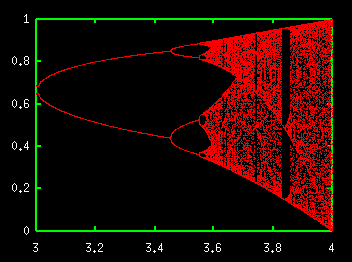# Bifurcation Diagram of Logistic MapLogistic map is a discrete time map written as xn+1=axn(1-xn). In spite of the simplicity of this equation, logistic map shows very complex behavior. So many studies have done. The above figure is the bifurcation diagram which appears when parameter a is varied. You can expand the above bifurcation diagram with the "Bifurcation diagram of the logistic map" simulator.

<< Attractors of Periodically Forced Pendulum / Bifurcation Diagram of Chaotic Neuron >>

Introduction to Chaos and Nonlinear Dynamics